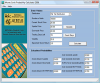Free Probability Calculator | Option Strategist
Home » Calculators » Free Probability Calculator

Calculate stock market probabilities with this easy to use program. Get more results using Monte Carlo simulation with McMillan's Probability Calculator Software.

## Inputs

Enter the following values:

Stock Price

Target Price

Calendar Days Remaining

Percent Annual Volatility
Stock volatility, where 25 = 25%. See our free volatility data section.

## Results

Below are the calculated probabilites:

Probability of stock being above Target Price    percent

Probability of stock being below Target Price    percent

# The Probability Calculator Software

### Simulate the probability of making money in your stock or option position.McMillan’s Probability Calculator is low-priced, easy-to-use software designed to estimate the probabilities that a stock will ever move beyond two set prices—the upside price and the downside price—during a given amount of time. The program uses a technique known as Monte Carlo Simulation to produce estimates that assess the probability of making money in a trade, but can also be used by traders to determine whether to purchase or sell stock, stock options, or combinations thereof.

#### What makes McMillan’s Probability Calculator different?

Over a number of trading days, the price of a stock may vary widely and still end up at or near the original purchase price. Many calculators are available that give the theoretical probability that a stock may approach certain values at the end of a trading period. In real trading, however, investors are following the price of a stock or stock options throughout the entire trading period. If the stock, stock options, or combination becomes profitable before the end of the trading period (for example, before the expiration of some stock options), it is reasonable that a trader may decide to reap part or all of those profits at that time. The Probability Calculator gives the likelihood that prices are ever exceeded during the trading period, not just at the end.